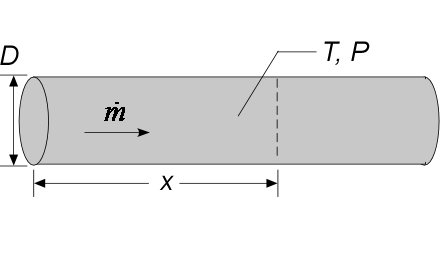Contents - Index

PipeFlow_localProcedure PipeFlow_local( Fluid\$, T, P, m_dot, D, x, RelRough: h_T_x, h_H_x,  dPdx) returns lower and upper bounds on the local heat transfer coefficient and the local pressure gradient at axial position x for a specified mass flow rate (m_dot) through a circular tube of diameter D and length L.  The pressure gradient is expressed in the EES pressure unit (Pa, kPa, bar, MPa, psi, atm) per m or ft, depending on whether EES is configured in SI or English units.  This procedure calls PipeFlow to obtain the integrated values.  Properties are evaluated at the bulk temperature T and pressure P.

Inputs:

Fluid\$ can be any fluid in the EES database.  The fluid can be an ideal gas or a real fluid.

T - the bulk temperature of the fluid at location x in  [C], [K], [F], or [R].

P -  pressure at location x in [Pa], [kPa], [bar], [MPa], [atm], or [psia].

m_dot  -  mass flow rate in [kg/s], or [lbm/hr]

D -  diameter of the tube in [m] or [ft]

x - position in the axial direction of the tube in [m] or [ft]

RelRough - the ratio of the dispersions on the wall of the tube to the tube diameter (must be between 0 and 0.05)

Outputs:

h_T_x  -  local heat transfer coefficient in [W/m^2-K] or [Btu/hr-ft^2-R] assuming that the pipe wall is at constant temperature (lower bound)

h_H_x  -  local heat transfer coefficient in [W/m^2-K] or [Btu/hr-ft^2-R] assuming a constant heat flux at the pipe wall (upper bound)

dPdx -  local pressure gradient.  [(Pa, kPa, bar,  or atm)/m] or  [(psi or atm)/ft] depending on EES pressure unit setting

Notes

This procedure  differentiates the average heat transfer coefficient and pressure gradient provided by procedure PipeFlow.

Two values of the local heat transfer coefficient are returned.  The first, h_T_x is determined assuming that the wall is at constant temperature.  The second (h_H_x) is determined assuming that the wall is subjected to a constant heat flux.  For laminar flow, these values should provide lower and upper bounds on the heat transfer coefficient.  For turbulent flow, these values are identical.  See PipeFlow or section 5.2 of Nellis and Klein for additional information.

Example

\$UnitSystem SI K Pa J

T=363 [K]                    "temperature of fluid in pipe"

P=101300 [Pa]          "pressure of air in pipe"

m_dot=0.05 [kg/s]    "flow rate"

D=0.15 [m]                   "pipe diameter"

x =5 [m]                         "position in pipe"

RelRough=0 [-]          "relative roughness"

call PipeFlow_Local('Air', T, P, m_dot, D, x,RelRough: h_T_x, h_H_x, dPdx)

{Solution:

dPdx=-0.7299 [Pa/m]

h_H_x=10.55 [W/m^2-K]

h_T_x=10.55 [W/m^2-K]}